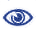# The Prague Stringology Conference 2009

Crochemore's repetitions algorithm revisited–computing runs

 Abstract: Crochemore's repetitions algorithm introduced in 1981 was the first O(n log n) algorithm for computing repetitions. Since then, several linear-time worst-case algorithms for computing runs have been introduced. They all follow a similar strategy: first compute the suffix tree or array, then use the suffix tree or array to compute the Lempel-Ziv factorization, then using the Lempel-Ziv factorization compute all the runs. It is conceivable that in practice an extension of Crochemore's repetitions algorithm may outperform the linear-time algorithms, or at least for certain classes of strings. The nature of Crochemore's algorithm lends itself naturally to parallelization, while the linear-time algorithms are not easily conducive to parallelization. For all these reasons it is interesting to explore ways to extend the original Crochemore's repetitions algorithm to compute runs. We present three variants of extending the repetitions algorithm to compute runs: two with a worsen complexity of O(n (log n)2), and one with the same complexity as the original algorithm. The three variants are tested for speed of performance and their memory requirements are analyzed. The third variant is tested and analyzed for various memory-saving alterations. The purpose of this research is to identify the best extension of Crochemore's algorithm for further study, comparison with other algorithms, and parallel implementation.

 Download paper:PostScript PDF BibTeX reference
 Download presentation: